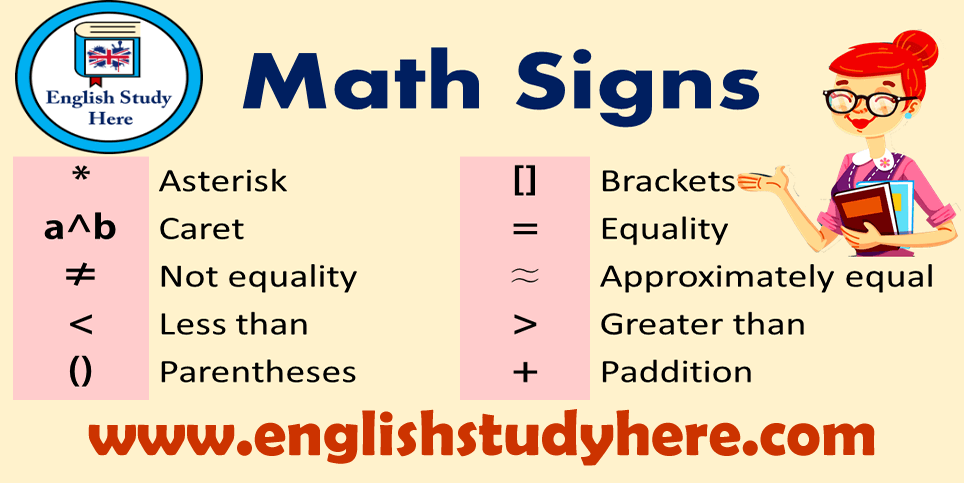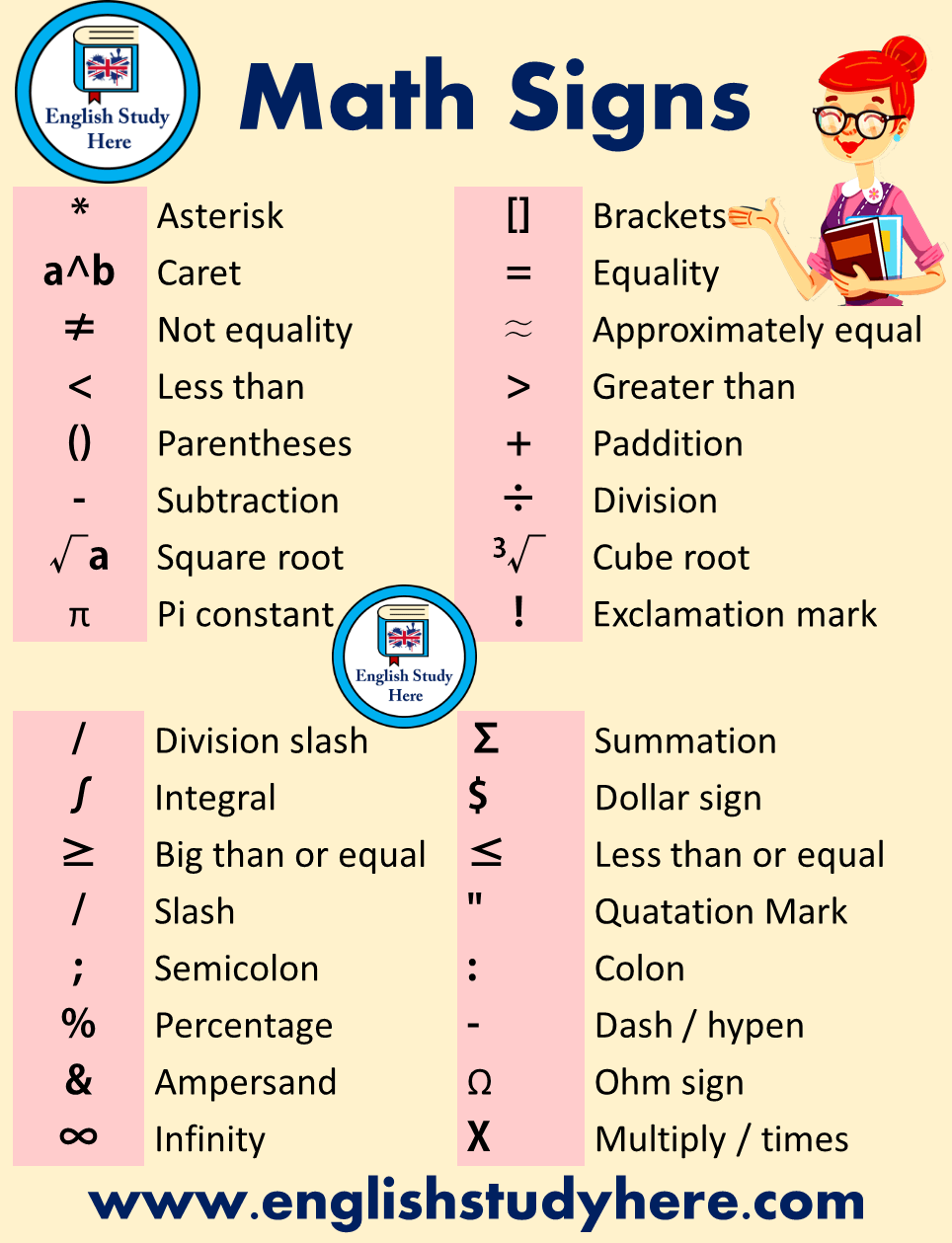# Mathematical Symbols List

Mathematical Symbols List, math signs in english;* Asterisk [] Brackets a^b Caret = Equality ≠ Not equality ≈ Approximately equal < Less than > Greater than () Parentheses + Paddition – Subtraction ÷ Division √a Square root 3√ Cube root π Pi constant ! Exclamation mark

 / Division slash Σ Summation ∫ Integral \$ Dollar sign ≥ Big than or equal ≤ Less than or equal / Slash “ Quatation Mark ; Semicolon : Colon % Percentage – Dash / hypen & Ampersand Ω Ohm sign ∞ Infinity X Multiply / times#### One Response

1.Ma.teresita dungog April 8, 2019